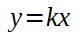# Representing Proportional Relationships by Equations

Instructor: Yuanxin (Amy) Yang Alcocer

Amy has a master's degree in secondary education and has taught math at a public charter high school.

After you read this lesson, you'll see just how easy it is to find an equation that represents your proportional relationship. You'll learn the formula you can use to find your equation for any proportion you have.

## Proportional Relationships

Imagine that you are the owner of an ice cream shop. Each day, you need to figure out just how many ice cream cones you sold and how much your total sales were. To do this, you use what is called a 'proportional relationship' between the number of ice cream cones you sold and how much you earned in sales.

A proportional relationship is where one ratio is the same as another ratio. For example, the ratio 1:2 is the same as 2:4. If you go ahead and divide both of these ratios, you'll get the same relationship 1:2. Another way of looking at it is you have to multiply the first number by 2 each time to get to the second number.

For your ice cream shop, if it costs \$1.40 for one ice cream cone, then it will cost \$2.80 for two ice cream cones because if you divide the \$2.80 by 2, you'll get the same result as when you divide your \$1.40 by 1. Both of them will give you \$1.40. To get to your price, you always multiply the number of ice cream cones by the same number, \$1.40.

So, in proportional relationships, you have to multiply one of the numbers by the same number each time to get to the second number.

## The Formula

Now comes the math. Once you know your proportional relationship, what you always multiply or divide by, you can use a formula to help you find your equation. This equation is a very easy one:• The y stands for your second set of numbers
• The x stands for your first set of numbers
• The k is your constant of proportionality.

The constant of proportionality is what number you need to multiply each number in the first column to get to the number in the second column.

For your ice cream shop, your constant of proportionality is what you always multiply with the number of ice cream cones to find how much you earned.

## Using the Formula

Now, let's see how easy this formula is to use.

Remember your ice cream shop costs. You can create a table with one column representing the number of ice cream cones sold and the second column of how much you earn.

Number of
ice cream cones
Amount earned
1 \$1.40
2 \$2.80
3 \$3.20
4 \$4.60
10 \$10.40
50 \$70.00

In order for you to use this formula, you'll need to find your constant of proportionality. To do this, ask yourself what number do you need to multiply each number in the first column to get to the number in the second column. Alternately, you can divide the second column by the first column.

To unlock this lesson you must be a Study.com Member.

### Register to view this lesson

Are you a student or a teacher?

### Unlock Your Education

#### See for yourself why 30 million people use Study.com

##### Become a Study.com member and start learning now.
Back
What teachers are saying about Study.com

### Earning College Credit

Did you know… We have over 160 college courses that prepare you to earn credit by exam that is accepted by over 1,500 colleges and universities. You can test out of the first two years of college and save thousands off your degree. Anyone can earn credit-by-exam regardless of age or education level.